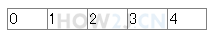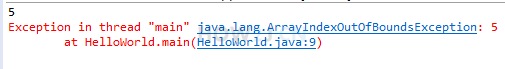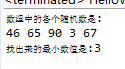8分35秒

## 声明数组

int[] a; 声明了一个数组变量。
[]表示该变量是一个数组
int 表示数组里的每一个元素都是一个整数
a 是变量名

public class HelloWorld { public static void main(String[] args) { // 声明一个数组 int[] a; } }
```public class HelloWorld {
public static void main(String[] args) {
// 声明一个数组
int[] a;
}
}
```

## 创建数组

new int

int c = 5; 这叫给c赋值为5

a = new int;public class HelloWorld { public static void main(String[] args) { //声明一个引用 int[] a; //创建一个长度是5的数组，并且使用引用a指向该数组 a = new int; int[] b = new int; //声明的同时，指向一个数组 } }
```public class HelloWorld {
public static void main(String[] args) {
//声明一个引用
int[] a;
//创建一个长度是5的数组，并且使用引用a指向该数组
a = new int;

int[] b = new int; //声明的同时，指向一个数组

}
}
```

## 访问数组public class HelloWorld { public static void main(String[] args) { int[] a; a = new int; a= 1; //下标0，代表数组里的第一个数 a= 2; a= 3; a= 4; a= 5; } }
```public class HelloWorld {
public static void main(String[] args) {
int[] a;
a = new int;

a= 1;  //下标0，代表数组里的第一个数
a= 2;
a= 3;
a= 4;
a= 5;
}
}
```

## 数组长度

.length属性用于访问一个数组的长度public class HelloWorld { public static void main(String[] args) { int[] a; a = new int; System.out.println(a.length); //打印数组的长度 a=100; //下标4，实质上是“第5个”，即最后一个 a=101; //下标5，实质上是“第6个”，超出范围 ,产生数组下标越界异常 } }
```public class HelloWorld {
public static void main(String[] args) {
int[] a;
a = new int;

System.out.println(a.length); //打印数组的长度

a=100; //下标4，实质上是“第5个”，即最后一个
a=101; //下标5，实质上是“第6个”，超出范围 ,产生数组下标越界异常

}
}
```

## 练习-数组最小值

姿势不对,事倍功半! 点击查看做练习的正确姿势

0-100的 随机整数的获取办法有多种，下面是参考办法之一:

(int) (Math.random() * 100)

Math.random() 会得到一个0-1之间的随机浮点数，然后乘以100，并强转为整型即可。
public class HelloWorld { public static void main(String[] args) { int[] a = new int; a = (int) (Math.random() * 100); a = (int) (Math.random() * 100); a = (int) (Math.random() * 100); a = (int) (Math.random() * 100); a = (int) (Math.random() * 100); System.out.println("数组中的各个随机数是:"); for (int i = 0; i < a.length; i++) System.out.println(a[i]); System.out.println("本练习的目的是，找出最小的一个值: "); } }
```public class HelloWorld {
public static void main(String[] args) {
int[] a = new int;
a = (int) (Math.random() * 100);
a = (int) (Math.random() * 100);
a = (int) (Math.random() * 100);
a = (int) (Math.random() * 100);
a = (int) (Math.random() * 100);

System.out.println("数组中的各个随机数是:");
for (int i = 0; i < a.length; i++)
System.out.println(a[i]);

System.out.println("本练习的目的是，找出最小的一个值: ");
}
}
```

## 答案-数组最小值3分49秒 本视频采用html5方式播放，如无法正常播放，请将浏览器升级至最新版本，推荐火狐，chrome，360浏览器。 如果装有迅雷，播放视频呈现直接下载状态，请调整 迅雷系统设置-基本设置-启动-监视全部浏览器 (去掉这个选项)。 chrome 的 视频下载插件会影响播放，如 IDM 等，请关闭或者切换其他浏览器public class HelloWorld { public static void main(String[] args) { int[] a = new int; a = (int) (Math.random() * 100); a = (int) (Math.random() * 100); a = (int) (Math.random() * 100); a = (int) (Math.random() * 100); a = (int) (Math.random() * 100); System.out.println("数组中的各个随机数是:"); for (int i = 0; i < a.length; i++) System.out.print(a[i] + " "); System.out.println(); int min = 100; for (int i = 0; i < a.length; i++) { if( a[i] < min ) min = a[i]; } System.out.println("找出来的最小数值是:" +min); } }

HOW2J公众号，关注后实时获知最新的教程和优惠活动，谢谢。2021-10-12 写方法写方法
 且远 关于 JAVA 基础-数组-创建数组 的提问 ```/** * @ClassName Arr * @Description 数组复习，找出数组中最小的数 * @Date * @Author * @Version 1.0 */ public class Arr { /** * @Description: 遍历并打印数组 * @Param: [arr] * @return: void * @Author: * @Date: */ public static void soutArr(int[] arr){ for (int i = 0; i < arr.length; i++) { System.out.println("arr = " + arr[i]); } } /** * @Description: 找出最小数 * @Param: [arr] * @return: int * @Author: * @Date: */ public static int minArr(int[] arr) { int min = Integer.MAX_VALUE; for (int i = 0; i < arr.length; i++) { if (min>arr[i]){ min = arr[i]; } } return min; } public static void main(String[] args) { int arr[] = new int; for (int i = 0 ; i < arr.length ; i++){ arr[i] = (int)(Math.random()*100); } soutArr(arr); System.out.println("minArr(arr) = " + minArr(arr)); } } ```

2021-10-04 代码
 鼎食 无4 个答案

Kion11

package cn; public class Text5 { public static void main(String[] args) { // TODO 自动生成的方法存根 int[] a=new int; int min; a=(int)(Math.random()*100); a=(int)(Math.random()*100); a=(int)(Math.random()*100); a=(int)(Math.random()*100); a=(int)(Math.random()*100); System.out.println("数组中的各个随机数是:"); for(int i=0;i<a.length;i++) { System.out.println(a[i]); min=a; for(int j=0;j<a.length-1;j++) { if(min>a[j+1]) { min=a[j+1]; } } System.out.println(min); } } }

2021-07-04 无从下手的话就添加一个最小的初始值，有了最小初始值这把大剑，一刀一个小朋友
2021-05-11 我的答案
2021-05-11 java.lang.ArrayIndexOutOfBoundsException: Index 5 out of bounds for length 5数组越界